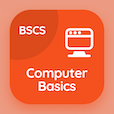Computer Science Courses Online

Digital Logic Design Prep Tests

Digital Logic Design Tests

The Book Introduction to Synchronous Sequential Logic Multiple Choice Questions (MCQ Quiz), Introduction to Synchronous Sequential Logic quiz answers PDF to study online courses, digital logic design tests. Practice Synchronous Sequential Logics Multiple Choice Questions and Answers (MCQs), Introduction to Synchronous Sequential Logic quiz questions for online software development courses. The eBook Introduction to Synchronous Sequential Logic MCQ App Download: clocked sequential circuits in dld, state reduction and assignment, flip-flops excitation tables, flip-flops in synchronous sequential logic test prep for online computer science schools.

The MCQ: How many types of sequential circuits have? PDF, "Introduction to Synchronous Sequential Logic" App Download (Free) with 2, 5, 6, and 7 choices for online software development courses. Study introduction to synchronous sequential logic quiz questions, download Google eBook (Free Sample) for computer software engineer.

## Logic Design: Introduction to Synchronous Sequential Logic MCQs

MCQ: How many types of sequential circuits have?

A) 2
B) 5
C) 6
D) 7

MCQ: Memory elements in clocked sequential circuits are called

A) latches
B) flip-flop
C) signals
D) gates

MCQ: Sequential circuits are

A) Synchronous
B) Asynchronous
C) signals
D) both a and b

MCQ: Synchronous sequential circuits that use clock are called

A) clocked sequential circuits
B) sequential circuits
C) logic circuits
D) complex circuits

MCQ: Classification of sequential circuits depends upon their timing of

A) feedback path
B) gates
C) signals
D) complex circuits

### Practice Tests: Digital Logic Design Exam Prep

Download Digital Logic Design Quiz App, Computer Basics MCQs App and DBMS MCQ App for Android & iOS devices. These Apps include complete analytics of real time attempts with interactive assessments. Download Play Store & App Store Apps & Enjoy 100% functionality with subscriptions!